## Friday, May 21, 2010

### Professor Quibb's Picks-2010

My personal picks for the 2010 Atlantic hurricane season:

18 cyclones attaining tropical depression status
17 cyclones attaining tropical storm status
7 cyclones attaining hurricane status
4 cyclones attaining major hurricane status

## Wednesday, May 19, 2010

### Hurricane Names List-2010

For the Atlantic Basin in 2010, the names list is as follows

Alex (used)
Bonnie (used)
Colin (used)
Danielle (used)
Earl (used)
Fiona (used)
Gaston (used)
Hermine (used)
Igor (used)
Julia (used)
Karl (used)
Lisa (used)
Matthew (used)
Nicole (used)
Otto (used)
Paula (used)
Richard (used)
Shary (used)
Tomas (used)
Virginie
Walter

This list is the same as the one used in 2004, except for Colin, Fiona, Igor, and Julia, which replaced the four retired hurricanes of 2004: Charley, Frances, Ivan and Jeanne.

## Tuesday, May 18, 2010

### Polytopes: Part IV

This is the final part of a four part post. For the first part, see here. For the second part, see here. For the third part, see here.

All the regular polytopes up through the fourth dimension have been discussed, and we couldn't directly visualize these elaborate structures, but we could understand their construction, and their polyhedral components. However, when one goes to the fifth dimension, all direct understanding is out of reach. And what of the sixth dimension? And the seventh? And the hundredth? How can we possibly deal with the polytopes in these dimensions? However, rather than increasing in complexity, regular polytopes become much simpler in higher dimensions, which allows us to generalize to n-dimensions in many aspects.

Starting with five dimensional space, we must consider a further extension of the Schlafli system. Since the structures in four dimensions were denoted by {p,q,r}, we now have five-dimensional polytopes {p,q,r,s}. Based on the sixteen regular polychora, ({3,3,3}, {4,3,3}, {3,4,3}, {3,3,4}, {5,3,3}, {3,3,5}, {5/2,3,3}, {3,3,5/2}, {5/2,5,3}, {5/2,3,5}, {5,5/2,5}, {3,5,5/2}, {5,3,5/2}, {5/2,5,5/2}, {5,5/2,3}, and {3,5/2,5}) one arrives at a staggering 34 possible forms:

{3,3,3,3}, {3,3,3,4}, {3,3,4,3}, {3,4,3,3}, {4,3,3,3}, {3,3,3,5}, {5,3,3,3}, {4,3,3,4}, {4,3,3,5}, {5,3,3,4}, {5,3,3,5}, {3,3,3,5/2}, {5/2,3,3,3}, {4,3,3,5/2}, {5/2,3,3,4}, {5,3,3,5/2}, {5,5/2,3,3}, {5/2,3,3,5}, {5,5/2,5,3}, {3,5/2,5,3}, {3,5,5/2,5}, {5,3,5/2,5}, {3,3,5,5/2}, {5/2,5,3,3}, {5,5/2,3,5}, {3,5,5/2,3}, {5/2,5,5.2,5}, {5/2,5,5/2,3}, {3,5/2,5,5/2}, {5,5/2,5,5/2}, {5/2,3,3,5/2}, {5/2,3,5,5/2}, {5/2,5,3,5/2}, {3,3,5/2,5}

11 of these involve only convex polychora, while the remaining 23 involve star polychora. Despite the vast range of possible forms, very few actually create polytopes in the fifth dimension. These are called 5-polytopes, or polytera (singular: polyteron). The curvature of these polytera is defined by determining whether the four dimensional solid angle around each vertex adds up to more than, less than, or exactly equal to, the four dimensional sphere. To find the curvature equation in higher dimensions, it becomes useful to use the general form of the equation in n dimensions. The function to find the formula is known as the (delta) equation. The curvature formula for a polytope {p,q,r...,y,z} (with any number of letters in between r and y) is expressed {p,q,r...,y,z}, and depends whether the resulting formula is greater than, less than, or equal to zero. The equation is defined recursively, or that each formula depends on the previous one counting up in dimensions. Assuming the trivial cases in one and two dimensions as follows (with {} implying the straight line as the universal polytope in one dimension):

∆{}=1 (this formula never changes in value, because all polytopes in one dimension are lines and are all basically identical)
and
∆{p}=(sin(π/p))^2 (this formula is always positive, corresponding to the fact that polygons always have positive curvature and are finite)

one can find the formula for any number of dimensions greater than two using:

{p,q,r...,y,z}={q,r,...,y,z}-{r,...,y,z}*(cos(π/p))^2

For example, to find ∆{p,q}:

∆{p,q}=∆{q}-∆{}*((cos(π/p))^2)=
(sin(π/q))^2-1*((cos(π/p))^2)=
(sin(π/q))^2-(cos(π/p))^2

By setting this greater than zero (solutions would then be finite polyhedra)

(sin(π/q))^2-(cos(π/p))^2>0
(sin(π/q))^2>(cos(π/p))^2
sin(π/q)>cos(π/p)

which, by a property of trigonometry, (for p,q>2, which, conveniently is what is required for true polyhedra) equals

sin(π/q)>sin(π/2-π/p)
π/q>π/2-π/p
π/p+π/q>π/2
1/p+1/q>1/2

The final formula seems very familiar, as it is the curvature formula from the second part of this post for polyhedra, that we have successfully derived using the formula! Using the same equation for {p,q,r,s} (I won't show all the work this time), we obtain the curvature formula for polytera. For a finite polyteron,

((cos(π/q))^2)/((sin(π/p))^2)+((cos(π/r))^2)/((sin(π/s))^2)<1

12 of the 34 total forms satisfy this: {3,3,3,3}, {3,3,3,4}, {4,3,3,3}, {3,3,3,5/2}, {5/2,3,3,3}, {4,3,3,5/2}, {5/2,3,3,4}, {5,5/2,3,3}, {3,3,5,5/2}, {5/2,5,5/2,3}, {3,5/2,5,5/2}, and {5/2,3,3,5/2}. However, all nine of these that are star polytera can be calculated to have infinite density, meaning that there are infinite planes in the polyteron. However, this is impossible in regular finite polytera, and therefore all but the first three can be eliminated. We will return to more general forms of {3,3,3,3}, {4,3,3,3} and {3,3,3,4} later.

There can also be tilings of Euclidean four dimensional space and these are the only ones that can be understood in four dimensions. The simplest example, {4,3,3,4}, also known as the tesseractic honeycomb, has four tesseracts (8-cells) at each face, and a three dimensional projection is shown below.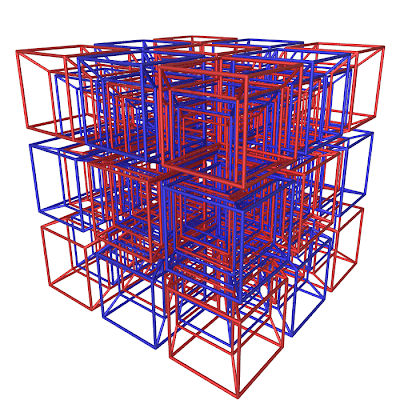With these figures, it is difficult to see any recognizable structure, but the 8-cells in this picture can vaguely be seen.

Similarly, {3,4,3,3} has three 24-cells at each face, and {3,3,4,3} has three 16-cells at each. No star polytera exist that are tilings of the Euclidean four dimensional plane, although {5,3,3,5/2}, {5/2,3,3,5}, {3,5/2,5,3} {3,5,5/2,3}, {5/2,5,5/2,5}, {5,5/2,5,2/2}, {5/2,3,5,5/2} and {5/2,5,3,5/2} all satisfy

((cos(π/q))^2)/((sin(π/p))^2)+((cos(π/r))^2)/((sin(π/s))^2)=1

However, all nine possible four dimensional hyperbolic tilings exist, namely: {3,3,3,5}, {5,3,3,3}, {4,3,3,5}, {5,3,3,4}, {5,3,3,5}, {3,5,5/2,5}, {5,5/2,5,3}, {3,3,5,5/2}, and {5/2,5,3,3} and all these satisfy

((cos(π/q))^2)/((sin(π/p))^2)+((cos(π/r))^2)/((sin(π/s))^2)>1

Finally, returning to the three finite regular polytera {3,3,3,3}, {3,3,3,4} and {4,3,3,3}, one can see that only four forms are possible in six dimensions: {3,3,3,3,3}, {3,3,3,3,4}, {4,3,3,3,3} and {4,3,3,3,4}. The last of these is a tiling (as we will soon see) and the only seven dimensional regular figures are {3,3,3,3,3,3}, {3,3,3,3,3,4}, {4,3,3,3,3,3} and {4,3,3,3,3,4}. The last of this is also a tiling, and the pattern continues. Therefore, for finite regular polytopes existing in n dimensional Euclidean space (n-1 dimensional elliptic space) there are only three forms:

{3^(n-1)}
The general polytope in n dimensions with n-1 3's in its symbol is known as the n-simplex. It has n+1 vertices and the rest of its elements, known as i-faces, come in numbers discussed below. The n-simplex is always a regular finite polytope in any number of dimensions.

Construction in n dimensions: Start with a point. This is the 0-simplex. Choose another distinct point and connect them. The result is a line segment, which is the 1-simplex. Choose a point outside of this line that is equidistant from the two existing points and connect them. The result is the regular triangle {3}, which is the 2-simplex. Choose another point outside this plane, that is equidistant from all three points and connect each pair of points with an edge. The result is the tetrahedron {3,3} which is the 3-simplex. Continue this procedure for any number of dimensions.

The number of i-faces (0-face=vertex, 1-face=edge, 2-face=face, 3-face=cell, etc.) in an n-simplex is based on the binomial theorem and Pascal's triangle. For an n-simplex, the number of i-faces is

(n+1)!/((i+1)!(n-i)!)

where ! is the factorial function (n!=1*2*3*4...*n).

For example, the number of faces on a 4-simplex is 5!/((3!)(4-2)!)=10, and the number of 5-faces in a 9-simplex is 10!/((6!)(9-5)!)=210.

The n-simplex is the simplest polytope that needs n dimensions to define, and is the general form of the sequence: point, line segment, triangle, tetrahedron, pentachoron... etc. In addition, n-simplices are most often represented by symmetric graphs that map out the vertices and show the connections between them. However, the drawback of this representation is that only vertices and edges can be mapped, and there is no easy way to see higher i-faces. As a result, symmetric graphs give few implications to the actual structure of the polytope. The symmetric graph of the 5-simplex {3,3,3,3} is shown below.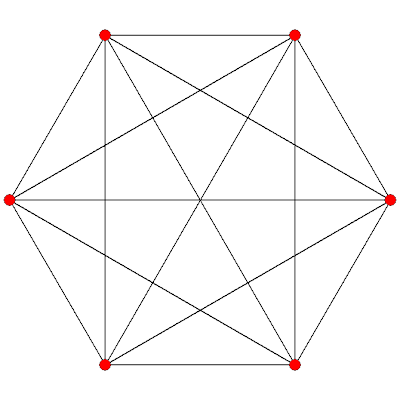The symmetric graph of the 5-simplex (or hexateron). The six vertices and 15 edges are visible, as is the fact that every pair of points is connected, but this view lacks any higher features. Also, for an n-simplex, the symmetric graph is always based on the regular polygon {n+1}.

{4,3^(n-2)}
The general polytope in n dimensions with a 4 followed by n-2 3's in its Schlafli symbol is known as the n-cube. It is always a finite regular polytope in any number of dimensions. It has 2^n vertices and the number of i-faces again depends on the binomial theorem but with an extra term in front of it. In general, to find the number of i-faces of a n-cube, the formula is

(2^(n-i))((n!)/((i!)(n-i)!))

For example, the number of edges (1-faces) on a 3-cube is (2^(3-1))((3!)/((1!)(3-1)!))=12, and the number of 7-faces on an 18-cube is (2^(18-7))((18!)/((7!)(18-7)!))=65175552.

Construction in n dimensions: To construct a regular n-cube, begin with a point. This is the 0-cube. Choose another distinct point and connect them. The result is a line segment, which is the 1-cube. Define another line segment as the shifting of the original one which is parallel to the original and so the distance between the lines is the same as the length of each line segment. Connect the corresponding vertices on the two line segments, and the result is the square {4}, which is the 2-cube. Take this square, and shift it out of the existing plane up or down the distance between any two points, to obtain two parallel squares. Connect each original point to its corresponding shifted point. The result is the 3-cube {4,3}. Continue for any number of dimensions to obtain any n-cube. To demonstrate this procedure, the construction for the 4-cube {4,3,3}, also called the 8-cell, is shown below.n-cubes, just like n-simplices, can be expressed with symmetric graphs. For example, the 6-cube's symmetric graph is shown below.The n-cube is also called the measure polytope in n dimensions, and is the general form of the sequence point, line segment, square, hexahedron, octachoron... etc.

{3^(n-2),4}
The general polytope in n dimensions with n-2 3's followed by a final 4 in its Schlafli symbol is known as the n-orthoplex, or the cross polytope. It is the general dual of the n-cube, and has 2n vertices. A general form for the number of i-faces is once again based on the binomial theorem. In general, the number of i-faces in an n-orthoplex is

(2^(i+1))((n!)/((i+1)!(n-(i+1))!))

For example, the number of cells in a 5-orthoplex is (2^(4))((5!)/((4)!(5-(5))!))=80, and the number of 6-faces in a 10-orthoplex is (2^(7))((10!)/((7)!(10-(7))!))=15360.

Construction in n dimensions: Start with a point. This is the 0-orthoplex. Choose another distinct point and connect the two. The result is the 1-orthoplex, or a line segment. Then choose two points equidistant from the existing two and connect them to the existing points in such a way that a square is formed (eliminate the line between the original two points). The square {4} is the 2-orthoplex. Then, choose a point equidistant from all four of the existing points that is the same distance from each point as each point is from another adjacent to it. Also choose this point's reflection through the square. Connect all points, except those directly opposite from each other. The result is the octahedron {3,4} which is the 3-orthoplex. This is a more complex construction process, but it is easy to see with a little thought that it produces the orthoplexes. This process can be continued for any number of dimensions.

As with the first two general polytopes, the n-orthoplex can be represented with a symmetric diagram. For example, the layout of the 7-orthoplex's 14 vertices into the regular polygon {14} looks like this: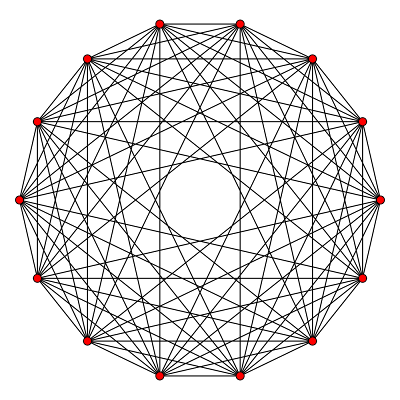This symmetric graph looks similar to that of a simplex, but with one exception. The vertices in a simplex are all connected in every possible way, but in an orthoplex, points are connected to every other point except the one directly opposite it. In conclusion, the n-orthoplex is a convex regular finite polytope in any number of dimensions, and is the general term of the sequence point, line segment, square, octahedron, 16-cell, etc.

{4,3^(n-2),4}
The fourth and final regular polytope in n-dimensions is represented by a 4, followed by n-2 3's, and then another 4. It is an infinite cubic honeycomb in Euclidean geometry, which is why {4,3^(n-2),4} needs only n dimensions to be visualized. If it were an elliptic or hyperbolic polytope, it would need n+1 dimensions, and the symbol would be {4,3^(n-3),4}. The elements of the n-cubic honeycomb are simply n-cubes.

After five dimensions, the above four are the only regular polytopes for each dimension, but there are many other polytopes with regular elements, and these are known as uniform polytopes. One subset of uniform polytopes is the regular ones discussed above, and another is the quasi-regular polytopes, which are based on two types of i-faces. There are limited possibilities for these, and these come in a few different families, each represented by a special Schlafli symbol {3^{a,b,c}}. Of the four families, only one provides an infinite number of quasi-regular polytopes. Note that the {a,b,c} doesn't tell the number of 3's, as before, but has a different meaning, which is not discussed here.

{3^{1,b,1}}
The first family of quasi-regular polytopes is represented {3^{1,b,1}}, where the variable b is used just to match up with the {a,b,c} above. These polytopes are known as n-demicubes. In n dimensions, the n-demicube is {3^{1,n-3,1}}. They are constructed by connecting alternating vertices of an n-cube with edges. To demonstrate this process, the 3-cube and 3-demicube are shown below.This image shows a transparent cube (3-cube) with its two possible demicubes. One connects alternate vertices, and the second simply connects all those not covered by the first. The two demicubes of a cube are always identical, and in this case, are two tetrahedra. Therefore, the tetrahedron, as well as being {3,3}, is also {3^{1,0,1}}. The n-demicube is quasi-regular beginning with the fifth dimension, as the 4-demicube is the 16-cell. Each n-demicube is made up of (n-1)-simplices and (n-1)-demicubes, and therefore is also defined recursively, with each demicube depending on the one before it. Each n-demicube has exactly 2n (n-1)-demicubes and 2^(n-1) (n-1)-simplices as (n-1)-faces. These are the highest faces before the polytope itself, and are sometimes called facets.

Since the 3-demicube is a tetrahedron, and the 3-simplex is also a tetrahedron, the 4-demicube is made of 8 tetrahedra and 8 tetrahedra, and the result is 16 tetrahedral cells, which is a 16-cell. However, the 5-demicube is made out of 10 4-demicubes, which are 16-cells, and 16 4-simplices, which are 5-cells, and the 5-demicube is therefore the first quasi-regular demicube. Continuing this pattern, one finds that there are infinite demicubes, all of which are finite and quasi-regular. As before, n-demicubes are also represented with symmetric graphs, and the 7-demicube is shown below in symmetric graph form.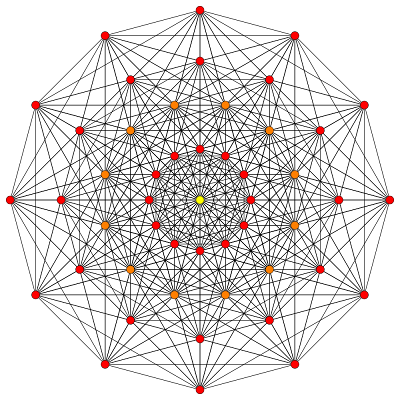The 7-demicube has only half the vertices of the 7-cube, but over 200 more edges. Also, red dots represent single vertices, while orange represent two overlapping and yellow four.

{3^{1,b,2}}
The second family of quasi-regular polytopes is the {3^{1,b,2}} family. The {3^{1,b,2}} polytope only is distinct from aforementioned polytopes when b takes the values 2, 3, 4 and 5, corresponding to polytopes in 6, 7, 8, and 8 dimensions, as we will see shortly. When b takes the value 0, the {3^{1,0,2}} polytope exists in four dimensions, and is simply {3,3,3}, or the 4-simplex. From there, the system is defined recursively, with each {3^{1,b,2}} in n dimensions (with one exception, see below) having {3^{1,b-1,2}'s and (n-1)-demicubes as facets. The polytope {3^{1,1,2}} has 4-simplices and 4-demicubes as facets, and this has already been discussed above as being the 5-demicube. Therefore,

5-demicube={3^{1,2,1}={3^{1,1,2}}

However, the {3^{1,2,2}} polytope has {3^{1,1,2}}'s and 5-demicubes as facets, which are both equivalent, and the {3^{1,2,2}} polytope is therefore a new polytope, made of 54 5-demicube facets. The next polytope {3^{1,3,2}} has {3^{1,2,2}}'s and 6-demicubes as facets, and the pattern continues. However, when one gets to {3^{1,5,2}} polytope, composed of {3^{1,4,2}}'s (in eight Euclidean dimensions, seven elliptic) and 8-demicubes, one finds not an elliptic polytope, but a new Euclidean tiling! Therefore, this infinite polytope also exists in eight dimensions. However, having a Euclidean tiling in the polytope family ends it, for if the {3^{1,6,2}} polytope existed, it would have to have {3^{1,5,2}}'s as facets, and these are infinite, which is not allowed in polytopes. Therefore, only four new polytopes arise from this family. The symmetric graph of {3^{1,3,2}} is pictured below.In this image, blue vertices are single and red have a multiplicity of 2 (having 2,4,6 or some even number of vertices coinciding).

{3^{2,b,1}}
The third of four families of quasi-regular polytopes is the {3^{2,b,1}} family. Again, only four new polytopes are generated by this family and these are when b=2, 3, 4, or 5. The facets of such a polytope in n dimensions (again, with one exception) are {3^{2,b-1,1}}'s and (n-1)-simplices. The {3^{2,0,1}} polytope is the 5-cell again and the {3^{2,1,1}} has 32 4-simplices, making it the 5-orthoplex. The first new polytope is {3^{2,2,1}} which has 5-orthoplexes and 5-simplices as facets. The pattern continues again until {3^{2,5,1}} which is another Euclidean tiling, made of an infinite number of 8-simplices and {3^{2,4,1}}'s. As before, the pattern must end there, since there cannot be a polytope with infinite facets, although a polytope can have an infinite number of facets. The {3^{2,4,1}} polytope is shown below.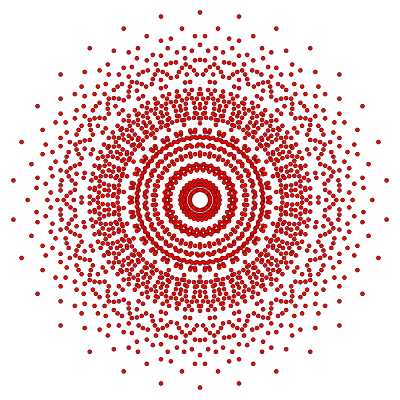This polytope exists in eight dimensional Euclidean space, and only its 2162 vertices are presented in this graph. Although it may seem that presenting only vertices doesn't present much of the polytope, the addition of edges would over clutter the image, as the {3^{2,4,1}} has over 69000!

{3^{a,2,1}}
The final quasi-regular polytope family is the {3^{a,2,1}} family and it is the only one of the four in which the first number a of the symbol varies and not the second, b. This family provides only 3 new polytopes in 5 dimensions and up, but yields a few interesting cases in lower dimensions as well. In n dimensions, the facets are simply (n-1)-simplices and (n-1)-orthoplexes. The sequence begins with the {3^{-1,2,1}} polytope, which exists in three dimensions and has triangles and squares as faces. The resulting figure is a triangular prism, which is simply two parallel triangles connected by three squares. The next polytope has 3-simplexes and 3-cubes as cells and is represented {3^{0,2,1}}. This polytope is equivalent to the rectified 5-cell. The next two, {3^{1,2,1}} and {3^{2,2,1}}, have been covered already. The first of these is the 5-demicube, and the second is discussed above and is a member of the {3^{2,b,1}} family.

The first distinct polytope is the {3^{3,2,1}} polytope which is made up of {3^{2,2,1}}'s and 6-orthoplexes. The pattern continues until, as before, the {3^{5,2,1}} polytope is a Euclidean eight dimensional tessellation with infinite {3^{4,2,1}}'s and 8-orthoplexes as facets. As before, {3^{6,2,1}} cannot exist as a result.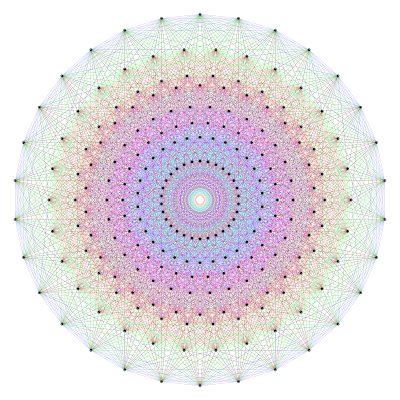The symmetric graph of the eight-dimensional (seven-dimensional elliptic) {3^{4,2,1}} polytope.

No other major families of quasi-regular polytopes exist, because all of the possibilities of recursive dependance, i.e. being based on the previous polytope and the simplex, or the orthoplex and simplex, etc. None involve square faces or any n-cubes at all, except of course, the n-cubes themselves, and are all based on triangles. However, there are many other uniform polytopes that may be obtained by operations on the three regular polytopes in n-dimensions. It has been discussed numerous times in the previous posts that truncation, or the slicing of vertices, and cantellation, or the slicing of edges. Also, in the previous part of this post, the idea of the slicing of cells in four dimensions and up, called runcination, was discussed. These operations can be extended, however, to the slicing of any i-face, and there are more possibilities in every dimension. For example, in five dimensions, the sterication operator, or slicing of 4-faces with respect to the fifth dimension, can be added to the four other operators, and any combination of the five can be applied. By the sixth dimension, there are hundreds of possibilities with the addition of the pentellation operator, and soon there are way too many to keep track of. Names of the polytopes also become unwieldy, as the combination of truncation, cantellation, sterication, and pentellation on a 6-simplex is named the pentistericantitruncated 6-simplex. Due to their diverse natrue, the uniform polytopes in seven dimensions and up have not been properly classified.

With any number of dimensions, and an infinity of possibilities in each, the world of polytopes is a limitless, beautiful branch of mathematics that defines much that we see in nature and what we find in abstraction.

Sources: Regular Polytopes by H.S.M. Coxeter, and various wikipedia titles: List of Regular Polytopes, k21 familiy, Uniform polyteron, Uniform polypeton, Truncation (geometry), etc.

## Sunday, May 9, 2010

### Polytopes: Part III

This is the third part of a four part post. For the first part, see here. For the second part, see here.

Our entire world, and our entire Universe, all that we know, is limited to the third dimension. How, then, can anyone understand the fourth? The fourth dimension, which eludes our visual understanding forever, can only be thought of mathematically. Yet so many wonders arise from it. In the fourth dimension, you can see the inside of a three-dimensional object. This is easily visualized by a lower dimensional analogy. Imagine two shapes on a two-dimensional plane. From their perspective, they can see only the outlines of shapes, and their inside remains hidden. However, someone in the third dimension looking from above could easily see the inside of such a shape, and identify it as a triangle, for instance. The same thing happens from the fourth to the third dimension. Other wonders include two infinite planes intersecting at a single point, and two cubes at an edge. To attempt to gain an understanding of this Universe's inhabitants, we must understand the structures in Euclidean 4-space. Although the actual structures are tilings of three dimensional planes, all but the tiling of the Euclidean plane can only be understood from the vantage point of the fourth dimension. The result is a higher class of polytope: the polychora (singular: polychoron).

Again extending the system used before, a regular polychora can be represented by {p,q,r}, which represents r polyhedra {p,q} at every edge. However, before one randomly assigns numbers for p, q, and r, one must take into account the restrictions provided by polyhedra. For {p,q,r} must be made up of finite polyhedra, and {p,q} must therefore be one of the nine regular polyhedra that existed previously. In addition, {q,r} represents the configuration around each vertex of the polychoron, called the vertex figure, and this must also be finite for the polychoron to exist. Taking all this into account, and using the nine regular finite polyhedra {3,3}, {3,4}, {4,3}, {3,5}, {5,3}, {5/2,5}, {5,5/2}, {5/2,3}, and {3,5/2}, one ends up with 25 possible regular polychora:

{3,3,3}, {4,3,3}, {3,4,3}, {3,3,4}, {4,3,4}, {5,3,3}, {3,5,3}, {3,3,5}, {5,3,5}, {4,3,5}, {5,3,4}, {5/2,3,3}, {3,5/2,3}, {3,3,5/2}, {5/2,3,5/2}, {5/2,5,3}, {5/2,3,5}, {5,5/2,5}, {3,5,5/2}, {4,3,5/2}, {5,3,5/2}, {5/2,5,5/2}, {5,5/2,3}, {3,5/2,5}, and {5/2,3,4}.

The curvature of these figures is determined by the measure of the solid angle around each vertex. The solid angle is an extension of the regular angle that exists in three dimensions. A "full" angle is a sphere surrounding a point, and a partial angle is one that only fills a portion of the surrounding sphere. An example of a solid angle is shown below.This angle, represented by the omega symbol, fills only a portion A of the entire sphere of radius r. It is apparent that r and A are proportional in this equation, because the size of A increases as r increases, but the value of omega remains the same. Note that the value "r" below is the value in {p,q,r}, not the radius of the sphere above.

The equation for the curvature of {p,q,r} is

((sin(π/p))^2)((sin(π/r))^2)>(cos(π/q))^2

which can be square rooted and adjusted to obtain

(sin(π/p))(sin(π/r))>cos(π/q)
(sin(π/p))(sin(π/r)-cos(π/q)>0

If this equation results in a value greater than 0, the polychoron is a tiling of elliptic three-dimensional space. As a result, they are finite, and can only be accurately expressed in Euclidean four-dimensional space. Six convex forms satisfy this formula, and five of these are extensions of the Platonic Solids. The polychora are constructed with polyhedra, and each polyhedron in a polychoron is known as a cell. The other elements are faces, edges, and vertices, just as before.

{3,3,3}
(sin(π/3))(sin(π/3))-cos(π/3)=((√3)/2)((√3/2))-1/2=3/4-1/2=1/4>0
The triplet {3,3,3} defines a convex regular polychoron with tetrahedra as cells with three at each edge. The resulting figure has five tetrahedral cells, ten triangular faces, ten edges, and five vertices. It is known as the pentachoron, or 5-cell. It is also the four-dimensional analog of the triangle and the tetrahedron. It is shown below.The above projection shows the wireframe Schlegel diagram of the pentachoron projected in the third dimension. This projection allows one to see each individual cell of a polychoron, although three of the five tetrahedra in the image are distorted, without being in the fourth dimension.Another projection of the pentachoron showing it as the tiling of a four dimensional sphere in elliptic geometry, The projection curves the faces and cells of the figure. This projection "unfolds" the three dimensional sphere onto a two dimensional plane, just like a flat map of the globe, and it is extended here to unfold the four dimensional sphere into a distorted three dimensional figure.

{4,3,3}
(sin(π/4))(sin(π/3)-cos(π/3)=((√2)/2)((√3)/2)-1/2=(√6)/4-1/2~.1123...
The triplet {4,3,3} defines a convex regular polychoron with cubes as cells and three at each edge. The resulting figure has eight cubic cells, 24 square faces, 32 edges, and 16 vertices. It is known as the octachoron, the 8-cell, or the tesseract. This polychoron is the four dimensional analog of the square and the cube. It is pictured below.The wireframe projection of the 8-cell. In the fourth dimension, all eight cubes are of equal size and shape, but they are distorted in this image.The elliptic projection of the 8-cell.

{3,3,4}
(sin(π/3))(sin(π/4)-cos(π/3)=((√3)/2)((√2)/2)-1/2=(√6)/4-1/2~.1123...
The triplet {3,3,4} defines a regular convex polychoron with tetrahedra as cells and four at each edge. The resulting figure has 16 tetrahedral cells, 32 triangular faces, 24 edges, and eight vertices. It is known as the hexadecachoron, or 16-cell. It is the four dimensional analog of a square and an octahedron. Note that the octahedron and cube are both based on the square, because the square is {4}, and the corresponding forms of the three and four dimensional analogs are {3,4}, {4,3}, {3,3,4}, and {4,3,3}, with a four at either the beginning or end of the symbol. WIth one dimension, these two cases are equivalent. because {4} with a 4 at the beginning is the same as {4} with a four at the end. The 16-cell is shown below.The wireframe projection of the 16-cell. As before, all 16 cells are the same size in the fourth dimension, but appear distorted when projected.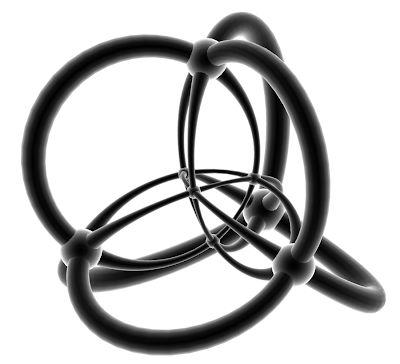Elliptic projection of the 16-cell.

{3,4,3}
(sin(π/3))(sin(π/3))-cos(π/3)=((√3)/2)((√3)/2)-(√2)/2=3/4-(√2)/2=.04289...
The triplet {3,4,3} defines a convex regular polychoron with octahedra as cells and three at each edge. The resulting figure has 24 octahedral cells, 96 triangular faces, 96 edges, and 24 vertices. It is known as the icositetrachoron, or the 24-cell. It is the only regular convex polychoron of the six that is not based on a lower polytope, and is my personal favorite (if I'm allowed to have one) of the six. It is shown below.The wireframe projection of the 24-cell. This is the only convex polychoron with octahedral cells.The elliptic 24-cell. This projection is the same as previous ones, except for the fact that each face of the figure is "bubbled".

{5,3,3}
(sin(π/5))(sin(π/3))-cos(π/3)=.00903696...
The triplet {5,3,3} defines a regular convex polychoron with dodecahedra as cells and three at each edge. The resulting figure has 120 dodecahedral cells, 720 pentagonal faces, 1200 edges and 600 vertices. It is known as the hecatonicosichoron, or 120-cell. It is the four dimensional analog of the pentagon and dodecahedron. The 120-cell is shown below.The wireframe projection of the 120-cell. Due to the projection, the polychoron is distorted so that the nearest cells actually appear to be the "smallest" and "farthest", but in reality the ones closest to the center, i.e. the smallest, are the closest from a four-dimensional viewpoint.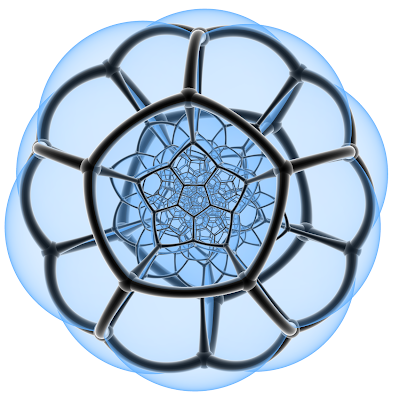Elliptic "bubbled" 120-cell. The same apparent paradox with the distorted viewpoint applies, as discussed above.

{3,3,5}
(sin(π/3))(sin(π/5))-cos(π/3)=.00903696...
The triplet {3,3,5} defines a regular convex polychoron with tetrahedra as cells and five at each edge. The resulting figure has 600 tetrahedral cells, 1200 triangular faces, 720 edges and 120 vertices. It is known as the hexacosichoron or 600-cell. It is the four dimensional analog of the icosahedron.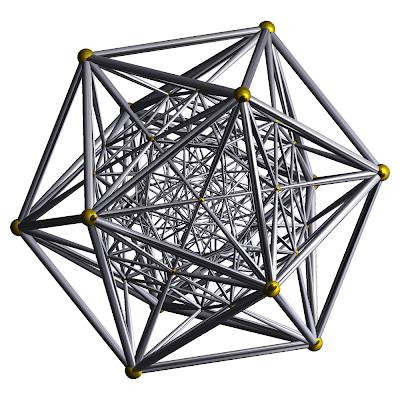The wireframe projection of the 600-cell.Elliptic projection of 600-cell. For both of the above projections the same viewpoint "paradox" that applies to the 120-cell applies here.

Many of the projections may be confusing, and this is because the cells closest in the four-dimensional viewpoint are bunched up in the center of the projection and are small. The reason for this can be easily seen with the projection of the dodecahedron into two dimensions.This is the projection of the dodecahedron onto a two dimensional plane. The faces "on top" are actually the nearest, while the bottom faces, i.e. the ones that would be the farthest away in three dimensions are on the edges. These are the largest, but must distorted.This image shows a similar wireframe projection of the 24-cell to the one shown above, but with the "closest" cell highlighted in red. This allows one to see that the closest cell is oriented in the center of the projection and is the least distorted.

Another way to show the polychora is by presenting their net. The net of a polytope is the "unfolded" polytope into its faces, if it is a polyhedron, or cells, if it is a polychoron.The net of the dodecahedron. If this net is folded into the third dimension, the pentagons line up as the faces of the dodecahedron.

The net of a polychoron is made of polyhedra. For example, the net of the 8-cell is eight cubes, as shown below. When this figure is folded into the fourth dimension, it becomes the 8-cell. For the nets of all of the six polychora above, see here.Of the six, four come in two dual pairs, the 8-cell and 16-cell, and 120-cell and 600-cell, and two are their own duals: the 5-cell, and the 24-cell. Also, for the six shown above, all have the property that the number of cells plus the number of edges is equal to the number of faces plus the number of vertices.

There are also non-convex regular finite polychora, but we will skip all that involve star polygons and therefore any star polyhedra as cells for now. Of the 25 cases above, 11 do not include the star polygon symbol 5/2. These are {3,3,3}, {4,3,3}, {3,4,3}, {3,3,4}, {4,3,4}, {5,3,3}, {3,5,3}, {3,3,5}, {5,3,5}, {4,3,5}, and {5,3,4}. Six of these have already been dealt with, (the regular convex finite polychora) and this leaves only five: {4,3,4}, {3,5,3}, {5,3,5}, {4,3,5}, and {5,3,4}. Using the formula above but with the case

(sin(π/p))(sin(π/r)-cos(π/q)=0,

where the curvature of {p,q,r} is zero, one can see that any solution to this equation would tile Euclidean three dimensional space. Only one of the above pairs fits that definition, and that is the only tiling of Euclidean three dimensional space, {4,3,4}. It is the only polychoron that most can truly visualize, because it can be expressed in three-dimensional Euclidean space, as opposed to the above polychora, which are in elliptic three dimensional space, and can only be truly expressed in Euclidean geometry with the use of four dimensions. {4,3,4} is shown below.

{4,3,4}
(sin(π/4))(sin(π/4))-sin(π/3)=((√2)/2)((√2)/2)-1/2=2/4-1/2=0This infinite polychoron has four cubes at each edge, and is also known as the cubic honeycomb.

The one remaining case of regular convex polychora is

(sin(π/p))(sin(π/r)-cos(π/q)<0

Four polychora satisfy this definition, {5,3,5}, {5,3,4}, {3,5,3}, and {4,3,5}. The formula states that these are negatively curved, and therefore are tilings in hyperbolic geometry. The first two have dodecahedra as cells, the third icosahedra, and the fourth, cubes. As an example, the case {3,5,3} is shown below.

{3,5,3}
(sin(π/3))(sin(π/3))-cos(π/5)=-.86803...This infinite polychora is a tiling of negatively curved space, or hyperbolic space. Each edge is surrounded by three icosahedra. The projection here is an extension of the bounding circle used in three dimensional models to a bounding sphere, which contains the entire hyperbolic universe in one finite sphere. As a result all of the icosahedral cells appear distorted but are all regular and of the same size from a hyperbolic viewpoint. The center icosahedron is highlighted to emphasize a cell. Other than this, however, the cells are hard to identify. The other three dimensional hyperbolic tilings are similar in structure to the above one.

14 polychora have not been discussed out of the 25 above. The remaining ones have at least one 5/2 in their symbols, making them non-convex star polychora. The 14 possible forms for regular star polychora are:

{5/2,3,3}, {3,5/2,3}, {3,3,5/2}, {5/2,3,5/2}, {5/2,5,3}, {5/2,3,5}, {5,5/2,5}, {3,5,5/2}, {4,3,5/2}, {5,3,5/2}, {5/2,5,5/2}, {5,5/2,3}, {3,5/2,5}, and {5/2,3,4}.

{3,5,5/2}
(sin(π/3))(sin(π/(5/2)))-cos(π/5)=.014622...
The triplet {3,5,5/2} defines a non-convex regular polychoron with icosahedra as cells, and five at each vertex, but with each cell penatrating the interior of the polychoron. The resulting figure has 120 icosahedral cells, 1200 triangular faces, 720 edges, and 120 vertices. It is known as the icosahedral 120-cell, and is shown below.The drawback of this projection is that the faces are not transparent, and it is therefore impossible to view the interior of the polychoron, as opposed to the wireframe view, where the interior is revealed. This polychoron appears convex, but is not in four dimensional space.

{5/2,5,3}
(sin(π/(5/2)))(sin(π/3))-cos(π/5)=.014622...
The triplet {5/2,5,3} defines a non-convex regular polychoron with small stellated dodecahedra as cells, with three at each edge. The resulting figure has 120 small stellated dodecahedral cells, 720 pentagrammic faces, 1200 edges, and 120 vertices. It is known as the small stellated 120-cell, and is pictured below.The solid cell projection of the small stellated 120-cell. A few pentagrammic {5/2} faces are clearly visible, but it is difficult to isolate those that penetrate the polychoron's interior.

{5,5/2,5}
(sin(π/5))(sin(π/5))-cos(π/(5/2))=.0364745...
The triplet {5,5/2,5} defines a non-convex regular polychoron with great dodecahedra as faces and five at each edge. The resulting figure has 120 great dodecahedral cells, 720 pentagonal faces, 720 edges and 120 vertices. It is known as the great 120-cell, and is shown below.The solid cell projection of the great 120-cell. The great dodcahedra are evident, along with the pentagons as faces. The easiest one to spot is in the front, and is colored light purple.

{5,3,5/2}
(sin(π/5)(sin(π/(5/2)))-cos(π/3)=.059010699...
The triplet {5,3,5/2} defines a non-convex regular polychoron with dodecahedra as cells and five at each edge, with some penetrating the interior of the polychoron. The resulting figure has 120 dodecahedral cells, 720 pentagonal faces, 720 edges, and 120 vertices. It is known as the grand 120-cell, and is pictured below.The projection of the grand 120-cell is exactly the same as that of the great 120-cell for reasons discussed below. However, the dodecahedra in this image are visible as well as the great dodecahedra, and one can spot two types of cells in this same image!

{5/2,3,5}
(sin(π/(5/2)))(sin(π/5))-cos(π/3)=.059010699...
The triplet {5/2,3,5} defines a non-convex regular polychoron with great stellated dodecahedra as cells, and five at each edge. The resulting figure has 120 great stellated dodecahedral cells, 720 pentagrammic faces, 720 edges, and 120 vertices. It is known as the great stellated 120-cell and is shown below.The solid face projection of the great stellated 120-cell. The pentagrammic faces and great stellated dodecahedral cells are easily identified.

{5/2,5,5/2}
(sin(π/(5/2)))(sin(π/(5/2)))-cos(π/5)=.0954915...
The triplet {5/2,5,5/2} defines a non-convex regular polychoron with small stellated dodecahedra as cells, and five at each edge, with each penetrating the interior of the polychoron. The resulting figure has 120 small stellated dodecahedral cells, 720 pentagrammic faces, 720 edges, and 120 vertices. It is known as the grand stellated 120-cell, and is shown below.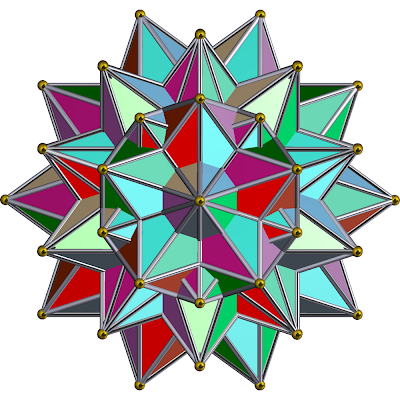The solid face projection of the grand stellated 120-cell, which is exactly the same as that of the great stellated 120-cell for the same reason as above, and it is discussed below.

{5,5/2,3}
(sin(π/5))(sin(π/3))-cos(π/(5/2))=.200019966...
The triplet {5,5/2,3} defines a non-convex regular polychoron with great dodecahedra as cells and three at each edge. The resulting figure has 120 great dodecahedral cells, 720 pentagonal faces, 1200 edges, and 120 vertices. It is known as the great grand 120-cell, and is pictured below.The solid face projection of the great grand 120-cell. The pentagonal faces are hard to spot, because they pass through the interior of the polychoron, but they are there, as are the great dodecahedral cells.

{3,5/2,5}
(sin(π/3))(sin(π/5))-cos(π/(5/2))=.200019966...
The triplet {3,5/2,5} defines a non-convex regular polychoron with great icosahedra as cells and five at each edge. The resulting figure has 120 great icosahedral cells, 1200 triangular faces, 720 edges, and 120 vertices. It is known as the great icosahedral 120-cell, and is pictured below.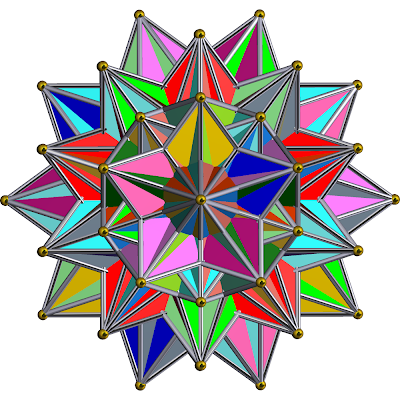The solid face projection of the great icosahedral 120-cell. The triangular faces are exceptionally hard to spot here, but they can be seen by connecting three points on opposite sides of the polychoron. The points of the great icosahedra can be seen, but their structure is hidden in the interior.

{3,3,5/2}
(sin(π/3))(sin(π/(5/2)))-cos(π/3)=.323639....
The triplet {3,3,5/2} defines a non-convex regular polychoron with tetrahedra as cells and five at each edge, but with each penetrating the polychoron's interior. The resulting figure has 600 tetrahedral cells, 1200 triangular faces, 720 edges, and 120 vertices. It is known as the grand 600-cell and is shown below.The solid face projection of the grand 600-cell. For the final time, this projection is identical to that of the great icosahedral 120-cell, despite having different numbers of cells.

{5/2,3,3}
(sin(π/(5/2)))(sin(π/3))-cos(π/3)=.323639....
The triplet {5/2,3,3} defines a non-convex regular polychoron with great stellated dodecahedra as cells, and three at each edge. The resulting figure has 120 great stellated dodecahedral cells, 720 pentagrammic faces, 1200 edges, and 600 vertices. It is known as the great grand stellated 120-cell, and is pictured below.The solid face projection of the great grand stellated 120-cell. The pentagrammic faces are fairly evident, as are the great stellated dodecahedra.

The ten above polychora come in four dual pairs:
Icosahedral 120-cell & Small Stellated 120-cell
Grand 120-cell & Great Stellated 120-cell
Great Grand 120-cell & Great Icosahedral 120-cell
Grand 600-cell & Great Grand Stellated 120-cell
and the remaining two, the Great 120-cell, and Grand Stellated 120-cell, are their own duals.

In addition, there are many similarities between the above polychora. In terms of vertex arrangement, the first nine polychora shown share the vertices of the 600-cell, and there are 120 of them, while only the tenth has 600 vertices and corresponds to the vertex arrangement of the 120-cell. In terms of edge arrangements, the 600-cell, the icosahedral 120-cell, the grand 120-cell, and the great 120-cell all share an edge and vertex arrangement, as do the small stellated 120-cell and the great grand 120-cell, the great stellated 120-cell, the great 600-cell, the grand stellated 120-cell and the great icosahedral 120-cell, leaving the great grand stellated 120-cell all by itself. Finally, there are three pairs above whose projections are identical because they have the same vertex, edges and face arrangement. Only the cells are connected differently, and this can only be seen in four dimensions. This applies to the great 120-cell and grand 120-cell, the great stellated 120-cell and the grand stellated 120-cell, and the great icosahedral 120-cell and the grand 600-cell.

The naming of these polychora comes from the processes used to create them. When one extends the edges of an existing polytope until it intersects itself (if it ever does) and forms another polytope, such as the pentagon to the pentagram, this is called stellation. Anything with "stellated" in its name has gone through this process. When the faces are extended from a polytope (polyhedron or higher) until they intersect themselves (if they ever do), and another polytope is formed, this is called greatening. Any polyhedron or polychoron with "great" in its name has undergone this process. Finally, if the cells (of a polychora or higher) are extended until they intersect themselves and form another polytope, this is known as aggrandizement. Any polychoron with "grand" in its name has undergone this process. These three processes are used to construct the four star polyhedra and 10 star polychora from the icosahedron and dodecahedron, and 600-cell and 120-cell, respectively.

After all these forms have been dealt with, there are only four possible pairs remaining: {4,3,5/2}, {5/2,3,4}, {5/2,3,5/2}, and {3,5/2,3}. These all have positive curvature, but none of them form regular star polychora. One can always begin to construct them, but they never close up and an infinite number of cells result, which is an impossibility in finite polychora.

All of the above deals with regular polychora, but one can also find polychora with more than one type of regular cell. These are known as uniform polychora. As with polyhedra truncation and cantellation are used to obtain these figures. However, since truncation is the slicing of vertices and can be used on polygons and higher, and cantellation is the slicing of edges and can be used on polyhedra and higher, there is a third operation, known as runcination. This process slices cells with respect to the fourth dimension, a process which is hard to understand from our third dimensional viewpoint.

When a polychoron is truncated, it turns from the ordinary {p,q,r} into what is written as t0,1{p,q,r}. The truncated 120-cell is shown below.Wireframe projection of the truncated 120-cell, where new cells are shown in red.

When a polychoron {p,q,r} is rectified, or truncated to the point that the original edges disappear it is written t1{p,q,r}. If the resulting polychoron is truncated again, it is bitruncated, and if it is rectified again, it is known as birectified. A polychoron {p,q,r} must be rectified three times before resulting in its dual, {r,q,p}. As a result, there are five intermediate steps between a regular polychoron and its dual when truncating that result in a uniform polychoron:

Parent: {p,q,r}
Truncated: t0,1{p,q,r}
Rectified: t1{p,q,r}
Bitruncated: t1,2{p,q,r}
Birectified: t2{p,q,r}, same as t1{r,q,p}
Tritruncated: t2,3{p,q,r}, same as t0,1{r,q,p}
Trirectified or Dual: t3{p,q,r}, same as {r,q,p}

With cantellation, there are two intermediate steps between a polyhedron and its dual:

Cantellated t1,2{p,q,r},
Bicantellated t2,3{p,q,r}, same as t1,2{r,q,p}

For example, the cantellated 8-cell looks like this:As before, new cells formed by the cantellation are shown in red and yellow.

With rucination, there is only one intermediate step, and it is the runcinated polychoron t0,3{p,q,r}. The rucinated 5-cell is shown below.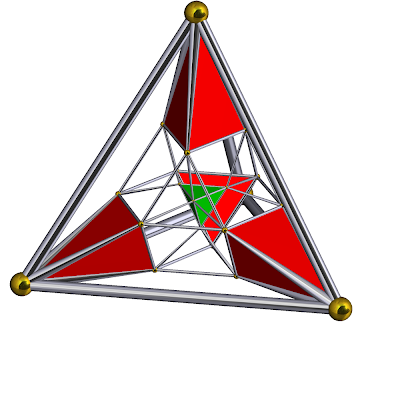This operation separates cells that were previously connected, and puts new cells in their place. This image shows the new cells from the rucination of the four distorted original cells in red, and highlights the new cell from the fifth, which makes up the tetrahedral envelope, or the outside of the figure, in green.

Finally, any combination of the above operators can be used. The most special case is when all three operators, truncation, cantellation, and rucination are all done to a polychoron. Then, the polychoron is omnitruncated. This is expressed t0,1,2,3{p,q,r}. As an example, the omnitruncated 8-cell, which is written t0,1,2,3{4,3,3}, is shown below.One can also apply these operations to infinite polychora, such as the cubic honeycomb {4,3,4}. For example, the truncated cubic honeycomb t0,1{4,3,4}, replaces cubes with truncated cubes, and fills the resulting spaces with regular octahedra. As a result, this uniform honeycomb still covers the entire Euclidean three dimensional space. A section of this honeycomb is shown below.The same processes can be applied to hyperbolic tilings as well.

In addition, there is an "inverse" of an omnitruncated polychoron, called a snub polychoron. It is creating by applying birectification to alternate vertices of a polytope. It is denoted s{p,q,r} for polychora. The snub 24-cell is shown below.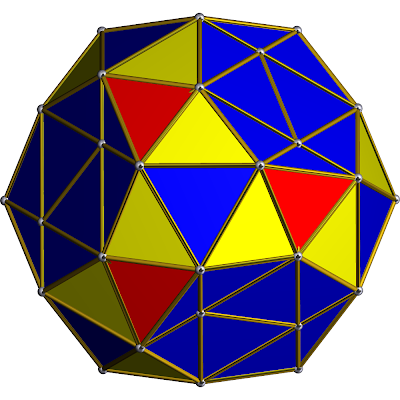This polychoron appears to have edges on flat faces, but in reality the flattened dark blue areas that are not triangular are distorted icosahedra. The structure of the snub 24-cell is more easily examined by use of its net, which is shown below.In the net of the snub 24-cell, the tetrahedra and icosahedra that make it are clearly visible.

The above operations on convex regular polychora plus their snubs make up 46 out of the 47 distinct convex uniform polychora. The 47th is a four dimensional prism that cannot be expressed with a Schalfli symbol {p,q,r}, and is unique in that aspect. It is made of tetrahedral and pentagonal antiprismic cells. The pentagonal antiprism is the polyhedron with three triangles and one pentagon around each vertex.The 47th uniform polychoron, or the grand antiprism, is a composition of 300 tetrahedral cells and 20 of the type above. It is the only irregular pattern in uniform polychora.

It has been proved that there are exactly 47 uniform convex polychora, but the total number of non-convex uniform polychora is not known, and there may be thousands! There are many polychora not based on regular ones, but these are not directly related to regular polytopes, and are not mentioned. The only exception to this is the grand antiprism, which is based on prisms which are not regular, but the polychoron itself is still uniform.

Sources: http://mathforum.org, http://weimholt.com/andrew/polytope.shtml, Regular Polytopes by H.S.M. Coxeter, a variety of wikipedia titles: Uniform Polychora, Schlafli-Hess polychoron, List of Regular polytopes, etc.

This post is finished in Polytopes: Part IV.

## Saturday, May 1, 2010

### Polytopes: Part II

This is the second part of a four part post. For the first part, see here.

The space in which all possible planes reside is the third dimension. In three dimensional space, one can define six directions: up, down, left, right, forward and back. The structures in this space are known as polyhedra (singular: polyhedron). Polyhedra are constructed with polygons, with a certain number of polygons at each vertex. Regular polyhedra have a certain number of identical regular polygons at each vertex. By extending the system from previously, these are denoted {p,q}.

One can construct the polygon {p,q} by having q regular polygons {p} at each vertex. As with polygons, situations with p≤2 are trivial, and q≤2 also has this property. This is due to the fact that any polyhedron {p,1} equals {p}, and any {p,2} is two overlapping polygons {p}, again trivially equaling {p}.

For any {p,q}, the total angular measure around each vertex is q internal angles of the regular polygon {p}. Since the internal angle of a regular polygon with p≥3 is (1-2/p)180º, the total angular measure around each vertex is (1-2/p)q180º. If this value is less than 360º, than the polyhedron curves inward, and eventually intersects itself, forming a finite polyhedron. Therefore, for a finite polyhedron,

(1-2/p)q180<360
(1-2/p)q<2
1-2/p<2/q
1<2/p+2/q
1/p+1/q>1/2

The final formula sets a limit on the number of possible finite regular polyhedra (assuming p,q>2), and only five pairs of {p,q}, where p and q are whole numbers, meet these requirements. These are called the Platonic Solids:

{3,3}
1/3+1/3=2/3>1/2
The above pair {3,3} defines a polyhedron with three triangles at each vertex. The resulting figure has four triangular faces, six lines, four vertices, and is known as the tetrahedron. It is pictured below.{3,4}
1/3+1/4=7/12>1/2
The pair {3,4} defines another polyhedron with four triangles at each vertex. The resulting figure has eight triangular faces twelve lines, six vertices, and is known as the octahedron. It is pictured below.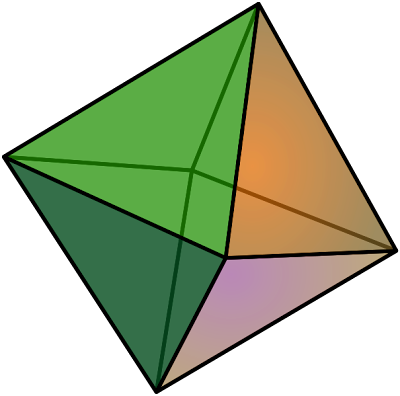{4,3}
1/4+1/3=7/12>1/2
The pair {4,3} defines another polyhedron with three squares at each vertex. The resulting figure has six square faces, twelve lines, eight vertices, and is known as the cube, or hexahedron. It is pictured below.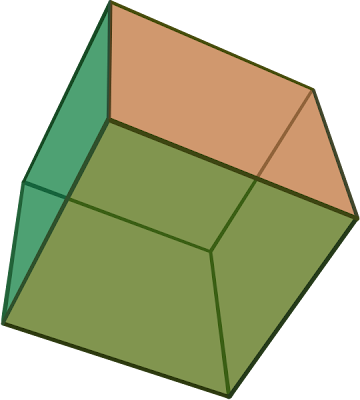{3,5}
1/3+1/5=8/15>1/2
The pair {3,5} defines yet another polyhedron with five triangles at each vertex. The resulting figure has twenty triangular faces, thirty lines, twelve vertices, and is known as the icosahedron. It is pictured below.{5,3}
1/5+1/3=8/15>1/2
The pair {5,3} defines a final polyhedron with three pentagons at each vertex. The resulting figure has twelve pentagonal faces, thirty lines, twenty vertices, and is known as the dodecahedron. It is pictured below.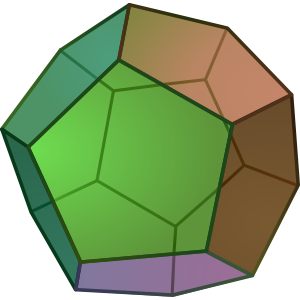The above polyhedrons are the only convex regular polyhedrons that are finite. They are finite due to the fact that they are tilings of a sphere, and therefore exist on the elliptic plane in elliptic geometry. Elliptic space is simply space with positive curvature. For more information on the three geometries, see here. In addition, convex regular polyhedra in elliptic 2-space or Euclidean 3-space (depending on point of view because elliptic and hyperbolic planes in n dimensions can only be accurately represented in n+1 dimensions) also have the property that the number of vertices plus the number of faces minus the number of sides always equals two. Also, for the above polyhedra, if the number of vertices and the number of faces are switched, the result is another polyhedron, known as the original polyhedron's dual. The dual of the tetrahedron is the tetrahedron, since both numbers are the same, and the other four come in dual pairs, with the cube's dual being the octahedron and the icosahedron's dual being the dodecahedron. This is equivalent to switching the numbers in {p,q} to {q,p}. As a result, if a polyhedron is finite, its dual will be also.

Another case to consider is when 1/p+1/q=1/2. In this case, the curvature is exactly zero, and the polyhedron tiles the Euclidean plane, and the polyhedron is known as a tiling. Since the flat Euclidean Universe is infinite, so are polyhedra that tile it. There are only three regular tilings of the Euclidean plane:

{3,6}
(1/6+1/3=9/18=1/2)The above are also known as the deltile, the quadrille, and the hextille, respectively.

The one remaining case, with 1/p+1/q<1/2,results in a tiling of negatively curved space, or hyperbolic space. It too, is infinite, and so are the polyhedra that tile it, but there are many projections of it into Euclidean geometry, and the one below squeezes the entire hyperbolic universe into one finite circle called the bounding circle.In the above picture, there are seven triangles at each vertex. The triangles appear to be distorted and of different size, but from the viewpoint of hyperbolic space, all triangles are the same size, and the circle is infinite.

There also can be non-convex polyhedra, for which the faces of the polyhedra penetrate their interior. However, of these, there are only four finite star polyhedra, known as the Kepler-Poinsot polyhedra, that are regular. The four are shown below.

{5/2,5}
1/(5/2)+1/5=2/5+1/5=3/5>1/2
The pair {5/2,5} defines a non-convex polyhedron with pentagrams as faces and five at each vertex. The resulting figure has twelve faces, thirty sides, and twelve vertices. It is known as the small stellated dodecahedron and is pictured below.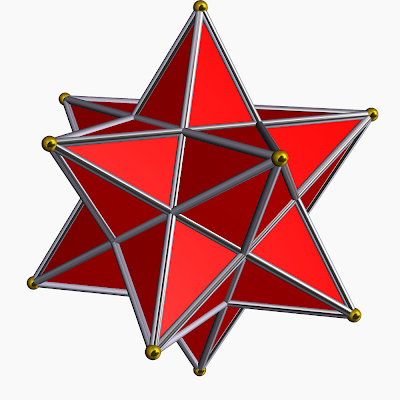{5,5/2}
1/5+1/(5/2)=1/5+2/5=3/5>1/2
The pair {5,5/2} defines a non-convex polyhedron with pentagons as faces and five at each vertex, although each cuts through the interior of the polyhedron. The resulting figure has twelve faces, thirty sides, and twelve vertices. It is known as the great dodecahedron and is pictured below.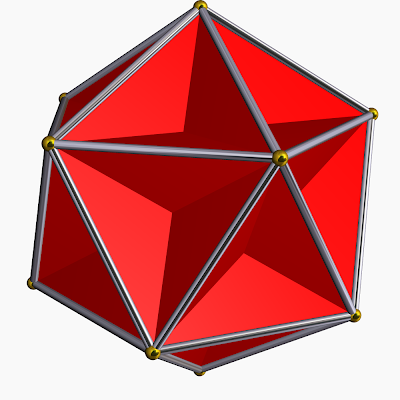{5/2,3}
1/(5/2)+1/3=2/5+1/3=11/15>1/2
The pair {5/2,3} defines a non-convex polyhedron with pentagrams as faces and three at each vertex. The resulting figure has twelve faces, thirty sides, and twenty vertices. It is known as the great stellated dodecahedron, and is pictured below.{3,5/2}
1/3+1/(5/2)=1/3+2/5=11/15>1/2
The pair {3,5/2} defines a non-convex polyhedron with triangles as faces, with five at each vertex, but each penetrating the interior of the polyhedron. The resulting figure has twenty faces, thirty sides and twelve vertices. It is known as the great icosahedron, and is pictured below.The four regular star polyhedra come in dual pairs with the small stellated dodecahedron and the great dodecahedron being one pair and the great stellated dodecahedron and the great icosahedron being the other. In addition, the vertices of each star polygon are in exactly the same orientation of one of the five Platonic Solids. The small stellated dodecahedron, the great dodecahedron, and the great icosahedron share the exact same vertex arrangement as the icosahedron, and the great stellated dodecahedron has the same arrangement as the dodecahedron. Also, the icosahedron and great dodecahedron have the same vertex and edge arrangements, and the small stellated dodecahedron and the great icosahedron form such a pair as well. However, in the first cases, the edges and faces are connected differently, and in the latter cases, just the faces. However, the star polyhedra are of less obvious construction than the Platonic solids, and it is often difficult to see the connections of the faces. For the sake of simplicity, a single face of each polyhedron is highlighted in yellow below to emphasize structure.Unlike the five regular convex polyhedra, the four regular star polyhedra by no means cover all the possibilities of the equation 1/p+1/q>1/2. In fact, {5/2,4}, {4,5/2}, {3,7/2}, {7/2,3}, {3,7/3}, {7/3,3}, {5/2,5/2}... etc. haven't been dealt with at all! To solve this problem, one must consider the fact that all of the regular star polyhedra share the vertices of a Platonic Solid just as a pentagram shares the vertices of a pentagon. Since no heptagons exist in the five regular convex polyhedra, any pair involving {7/2}, {7/3} or any higher star polygon must be disregarded. Also, star polyhedra are based on the extensions of faces on the five Platonic Solids. However, for the tetrahedron, octahedron, and cube, extending their faces into planes will not allow additional intersections, while the icosahedron {3,5} and dodecahedron {5,3} do. This eliminates any polytopes with a 4, or any number greater than 5, because the icosahedron and dodecahedron do not have these numbers. This leaves only the four already shown, and {5/2,5/2}, but it can be shown that the midpoints of the edges of {5/2,5/2} are equivalent to the vertices of {5/2,4}, and this last possibility is discarded. One can always begin to construct these star polyhedra, but they will never close up in a finite time, and require infinite sides and faces, which is impossible in the elliptic plane.

A similar method states that there can be no tiling of the Euclidean plane with star polygons, despite there being possible pairs, such as {10,5/2}, because they are not based on the three existing Euclidean tilings, {3,6}, {4,4} and {6,3}. Since no continuous star polygon has 3, 4, or 6 sides, no star polyhedron can be based on these numbers. There do, however, exist an infinite number of hyperbolic star polygon tilings, such as {11/2,5}, and many others.

The above explores all possible regular polyhedra. However, there are other polyhedra with regular faces, but not necessarily made of just one regular polygon. Some are composed of regular triangles and squares, for example. These are called uniform polyhedra. To generate these figures, one can apply processes such as truncation and cantellation to regular polyhedra. Truncation, when applied to the polyhedron is the process of taking away a vertex and putting a face in its place, while cantellation is taking away a edge and replacing it with a face. Both processes as performed on the cube are shown below.For the top picture, the slicing of the vertices creates triangles. When the vertices are cut to the point that the resulting faces intersect each other, but do not overlap, the polyhedron is rectified. The rectified cube is also known as the cubeoctahedron, which is last in the top row of pictures. If the cuboctahedron is truncated again, however, the resulting figure is the octahedron, which is the cube's dual. With cantellation, a slice is taken with respect to the edges. If the cube is half cantellated, as with the third picture in the second row, so that each polygon face is regular, the polyhedron is uniformly cantellated, and the resulting figure is known as the rhombicuboctahedron. If the polyhedron is cantellated to the point that the original faces disappear, the resulting figure is the original polyhedron's dual, in the case of the cube, the octahedron.

Using the symbols mentioned previously, fully truncated polyhedra are represented by t1{p,q}, where {p,q} is the regular polyhedron, also known as t0{p,q}. When truncated twice, the notation is t2{p,q}, but when a polyhedron is truncated twice, it becomes its dual, and therefore

t2{p,q}={q,p}

t0,2{p,q} is also the notation of the uniform cantellation of the polyhedron {p,q}.

Any regular polyhedron can undergo truncation and cantellation, including the star polyhedra. For example, the uniform truncation of a small stellated dodecahedron, as shown above, is simply a dodecahedron, because it is evident to see that if each point of the small stellated dodecahedron was cut off, there would only be pentagons left, and the figure would be a dodecahedron. If the small stellated dodecahedron is rectified, however, represented by t1{5/2,5}, the resulting figure is a dodecadodecahedron (a wonderful name) which is shown below.In general, any polyhedra made up of two types of polygons, {p}, and {q}, and with the ordering around each vertex goes {p}, {q}, {p}, {q}, are called quasiregular polyhedra, which are expressed by {p;q} (p semicolon q). For example {3;5} is a polyhedron that has two triangles and two pentagons at each vertex, which is known as the icosidodecahedron.In this figure, the pentagons and triangles alternate around each vertex.

In addition, a polyhedron {p,q} can always be found so that one of its truncations or cantellation results in any quasiregular polyhedron. Using the example above, the icosidodecahedron is the rectified dodecahedron. It is known to be finite because the angular measure around each vertex is 336º (108º+108º+60º+60º, which correspond to the angles of regular pentagons and triangles respectively), and this is less than 360º, making the polyhedron elliptic and finite, as a familiar formula from above states. Tilings of the Euclidean and hyperbolic planes can also be truncated and cantellated, and new tilings such as {3;6} and {3;7} arise from these processes. Both tilings, one the trihexagonal Euclidean tiling, and the triheptagonal hyperbolic tiling are shown below.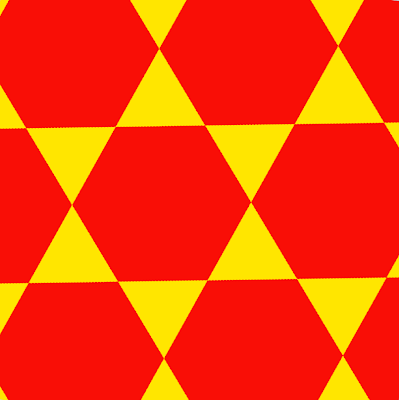These tilings are only two of the many Euclidean and infinite hyperbolic quasiregular tilings.

There are infinite additional polyhedra that do not have regular polygonal faces, and vary from square-based pyramids to hexagonal prisms. However, these polyhedra are not relevant to this post, and are therefore omitted.

Sources: Wikipedia Titles: List of Regular Polytopes, Uniform Polytope, Quasiregular Polytope, etc.
Regular Polytopes by H.S.M. Coxeter

Notation Note: In the above post, the words side, edge, and line are generally interchangeable, all representing the one dimensional features of a polyhedron.

For the next part of this post, see Polytopes: Part III.